How Discrete Event Simulators work

Let's assume you develop some kind of software system whose internal state evolves over time. You want to let users explore how the system evolves in different scenarios to help understanding the system or to perform some kind of analysis. So you have to write a simulator.

Let's be a bit more formal about what we want to do. Let's say our system state consists of a number of variables v1 ... vn. In practice, these probably aren't a flat list of variables, they might be structured in some way, as vectors, matrices, trees or whatever, but for the purpose of this article we'll keep it simple: we treat the system state as a set of variables vi. We use the term s (for state) to represent the set of all these variables.

Usually, some of these variables are connected through a set of system-specific rules. For example, v2 might be computed from v7 by dividing v7 by 3. Or v4 is always the sum of v2 and v1. Often, the current state will be computed by taking into account the state from various times previously; for example, the current v5 might be computed from a previous (yes, that's still vague, will get to that!) v5 plus the current v3. So our system really contains a model m (in the scientific sense) of the world we want to simulate. In our example where we can say

model {

state

v1 = 0

v2 = 10

v3 = 7

v4 = 0

v5 = 0

v6 = 0

v7 = 0

calc

v2 = v7 / 3

v4 = v1 + v2

v5 = v5@prev + v3

}

The purpose of the simulator is to let us explore how the model evolves over time, m(t). More specifically we want to know how the model state evolves, s(t), so we have to run the model for every t within the range we are interested in. Now, the interesting question is: for which ti do we have to run this? Are we really interested in s(t) for all t, or maybe only for some? How we answer this question drives the design of the simulator. I will explore three different options.

# Continuous

The first option is relevant for systems where the state evolves continuously. Conceptually we have to compute the state for every t.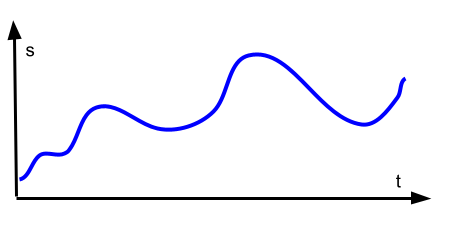Since time in the real world is continuous, this would result in an infinite number of computation steps. So we have to discretise time. How finely grained our time discretisation should be is not so easy to answer -- it depends on the precision requirements and sometimes the model itself (I will provide an example for this later).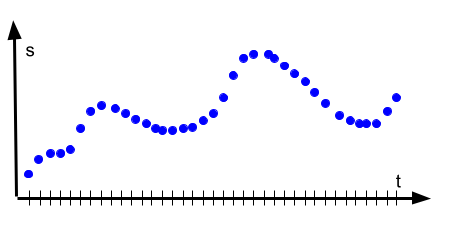The typical example for such a model is a weather or climate model. The state tracked by the model is temperature, dew point, cloud cover and the like for every point in the atmosphere within a particular region (say, Germany) with a particular resolution (say, 3 km horizontally and 100 m vertically). The calculations in the model are basically the physical laws -- or approximations thereof -- that govern how "weather" evolves over time: as cloud cover increases, temperature reduces; once we have enough clouds, temperature near the ground lowers far enough for there to be fewer thermals, resulting in a reduced cloud cover. And so on. Since we are really interested in the weather at any time during the forecasted period, we have to run it for every (discretized) t, say every 10 minutes -- this would be an example of a requirement driving the granularity of t. An example of the model itself driving the granularity would be that certain physical phenomena, such as turbulence and detailed cloud formation, cannot be calculated if the temporal resolution is too coarse. But I digress.

The point is: you write such a simulation basically by running a loop over time:

for (int t = 0; t < t_max; t += t_granularity) {

s[t] = runModel(m, s[0 .. t - 1])

}

The mechanics of the simulator are simple to implement (the complexity is in the model itself!) but it also has a huge drawback: it is expensive to compute, because you have to run the model for every t. For realistically fine-grained granularities of t, this can result in lots of model calculations.

# Schrödinger's Approach

Is the cat dead or alive? We don't know unless we look! This is another way of running a model: we only compute the state for those points in time when the user explicitly looks at the state. Let's say the user, for whatever reason, is only interested in the state of the model at times t = {7, 20, 33}. Can we make do with just three model runs, for t = 7, t = 20 and t = 33?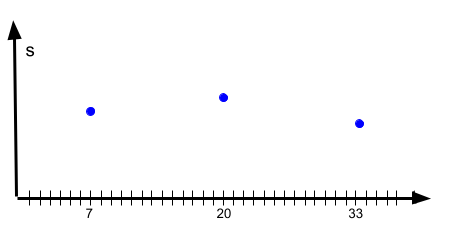There are two different cases here. One case is that the underlying system is still fundamentally continuous -- like the weather. If we decided we wanted to know the weather tomorrow at noon (let's say that is t = 36), then we still have to to run the whole model at its predefined granularity. The physics dictates that you have to evolve the weather step by step. Stephen Wolfram calls this computationally irreducible: there's no shortcut to running the calculations step by step. There's no "analytical" solution for weather(t). In this case we are back to the continuous  case above: we run the big loop until we reach t = 36 and then decide to just look at t = 7, t = 20 and t = 33. Nothing gained.

However, let's say we have this model:

model {

state

v1 = 0

v2 = 0

v3 = 0

calc

v1 = lookupV1(t)

v2 = v2 + t * 17

v3 = someFun(v2)

}

Note that here we are not calculating based on "previous" values of the model, we are just computing a new state based on actual functions. In this case, we can just plug in t = 7, t = 20 and t = 33 to get our three results. We don't need the states for the other t. We don't look, so the state doesn't need to exist.

This is of course much more efficient computationally. It's also trivial to implement. However, many systems -- weather! -- just don't work that way, the model doesn't allow the simplification. There's another problem: you have to know when to look. You have to know somehow when some interesting change happens so you can make the effort to look (and hence, calculate the model). For example, in our diagram here, we might get the impression that s remains between s1 and s2, while, in reality, it leaves these bounds during times we don't look (at t = 22 s is much bigger than s2). We just didn't know that something interesting might happen between t = 20 and t = 33.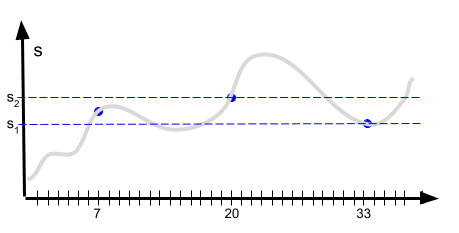It's not always clear that the user has a means of making the decision when to look. If they have -- for example, based on constraints outside the model -- and if the model allows it, then this approach is great because it is computationally very efficient.

For the intermediate times -- assuming that for some reason we can't or don't want to run the model -- we assume that the state stays constant, or that it evolves based on some other simple extrapolation (eg., the average between two adjacent known states).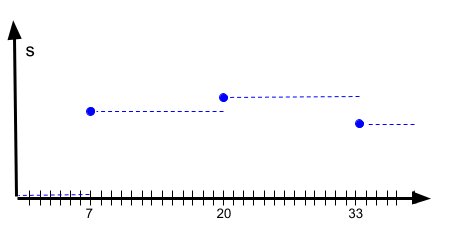There's a slight variation for the Schrödinger approach. Let's say a state change s1 to s2 should happen at t = 25. But the user only "looks" at t = 33. This means that between 25 and 33 no state update has happened, the state remains s1. When the user looks at t = 33 and we can update the state, we can do two things:

• we can make the state change to s2 at t = 33. This means that the change is delayed relative to what has happened in reality, but we don't rewrite the history for between t = 25 and t = 33
• alternatively we can retroactively register the state change to t = 25. This means that the state change is registered for the correct time, but we have just rewritten history; because in fact, between t = 25 and t = 33, while we hadn't looked the state was s1. If we look after back from the perspective of t = 33 the system claims that it has been s2.

In the end, to really be correct, we'd have to use bitemporal data storage.

# Entering User Interactions

Staying with our weather example, let's play god. God can modify the weather. Or maybe more practically, let's play climate scientist, and we want to simulate the eruption of a volcano. So we run our climate model for a year, with, say, a daily granularity. As we have said, this might not allow us to model certain finer-grained processes based on the actual physics (for example cloud formation during a day), but let's assume we have ways of approximating this reasonably (we change the model to use the approximations instead of the real-physics-formulas). So we are happily running our big loop, step by step, and now, at t = 666, we want to inject a volcano eruption. This is feasible to do in both approaches we have seen so far. We stop the simulation, and just "patch" the state of the model. Potentially, if the model makes calculations based on history, we might have to patch not just s but also, for example, s to s, but once we have made the patch, we can continue the simulation regularly. We'll come back to this later.

# Discrete Event Simulations

We have seen two ways of running models so far. The first one calculates the state for every t, given some previously decided granularity. It is precise (up to the granularity), but also expensive, because we have to execute the model lots of times. The second approach runs the model only at specific, user-decided times -- assuming the model's calculation logic allows it. This is very efficient (we run the model only a few times), but we might be overlooking interesting stuff between the times we look. Whether this is a problem for the particular simulation depends on the domain. It might not be, and then this approach is perfect.

There is a third option that lives in the middle between these two extremes. We run the model only for discrete times (and not for every t), but we also won't miss interesting things just because we didn't look. It's called a discrete event simulation, DES for short.

Note: There's a full implementation of a DES framework available for playing around with the idea. It's written in Kotlin: https://github.com/markusvoelter/KotlinDES. It has lots of comments that should connect well to the this article.

The core idea is that there is a queue of events. Every event in the queue is associated with a time tevent, and the queue is ordered by ascending time. Each event also contains instructions how to change the state at time tevent when the event is executed. This is similar to our god-like user interaction we have introduced above. We then run the model for tevent to bring the overall state in sync with the change we just made. The top-level loop looks basically as follows:

while (queue.isNotEmpty) {

event = queue.get()

t = event.time

event.modifyState(s[t])

runModel(m, s)

}

There are two ways events get into the queue. One is driven by the user. Through some means outside of the model we just know when to look or when to change the state explicitly. For example, in a simulation that tracks how a medical treatment evolves over time, we might decide to look at the state of the simulation every day at 08:00, 12:00 and 19:00 hours. Assuming we want to run the simulation for two weeks, we populate the queue with a just-look-and-dont-do-anything-Event for each of the 14 days at the three wall-clock times just mentioned. Or we just "know" that on day 3, 15:00 hours the simulated patient took a particular pill. So we add a PatientTookPill event for 3@1500.

The other way events enter the queue is through the simulation itself. Let's say that we want to understand how a fever is reduced in our simulated patient after the patient takes some medicine on day three at 15:00. The user inserts an event into the queue for 3@1500; the instruction part of that event changes the state of the system by making the simulated patient take their pill. In addition, it inserts additional events into the queue, to track how the patient's temperature changes over the next two hours: say for 3@1530, 3@1600, 3@1630 and 3@1700. Assuming the patient's temperature is one of the state variables, then just "looking" at the system will run the model at these four times and update the patient's temperature.

The previous case inserted events explicitly as part of the PatientTookPill event's instructions. But you can also register monitors that produce events if the state changes in a particular way. For example, we might want to say that whenever a patient's temperature goes above 37,5 °C (the fever threshold), then we want to automatically track the evolution of their temperature on an hourly basis for the next two days. We don't care how or why they develop a fever. It could be through a user "patch" or as a consequence of the model, but we definitely want to track it. You could put this event-creation logic directly into the model, but I find it more understandable and extensible to explicitly introduce the notion of a  model monitor. Here's the one for the fever:

if (justbecameTrue[t, s.temperature > 37,5]) {

for i in 1..96 {

add Event new JustLookEvent() at t + (i * 30 minutes)

}

}

}

So, to summarize, a DES computes the state for all user defined times plus for all those that are decided by the simulation itself. Like in the Schrödinger case, we assume that the state does not change between subsequent events (technically the state cannot change because that change would have to be caused by an event, but our assumption is that the system we are simulating -- the real world -- also doesn't change). Our system must not be continuous; our weather simulation wouldn't work as a DES.

There are two additional complications. The first one is that you can potentially run into loops where one event triggers a state change which, through monitors or other mechanisms, changes the state back (potentially indirectly). Your queue will never become empty, your main loop runs forever. If this happens you might have made a programming mistake. Or, alternatively, the system you're simulating just works that way. In order to prevent your simulation from running forever, you have to resolve the issue through fixpoint detection, vby limiting the number of change-createEvent-loops or by specifying a maximum number of simulation steps at which the simulation is stopped.

As mentioned above, the queue is ordered by time. This ensures that things happen in the order they should. However, there is a slight problem. What happens if multiple things happen at the same time? Let's be more precise about what this means. Let's say we are at time t = 100. An event's instructions lead to producing another event that should happen immediately. Right after the current one, but at the same "time" t = 100. To make this possible, we have to distinguish between the logical time (aka wall clock time) and a more dense time we use for execution. How can we achieve this?

• One option is to build your queue in a way where for each wall clock time ti there's an ordered list of events; when you schedule something to ti, the new event is appended to the end of the event list for time ti. The problem is that this makes your data structure more complicated and larger.
• The second option is to make your system's time a pair of {clock,dense} values. So if we want to perform an event right after t = 100, it would be schedule it to {100,1}. The next one scheduled for t = 100 would be scheduled to {100,2}. Your queue is sorted with a two-level sort key consisting of the two parts of the pair.
• The previous approach has the drawback that, whenever you insert something intot he queue at, say, t = 100, you first have to check whether there's already something for {100,0} or {100,1} or whatever, in order to correctly increment the dense time. It is therefore much easier to make the dense time a globally increasing counter. You basically count the events. So if you insert five events into the queue at t = 100, t = 101, t = 101 (same!), t = 110 and t = 200, they would be inserted as {100,0}, {101,1}, {101,2}, {110,3} and {200,4}; the dense time is just counting up. Since you're only eer looking at the dense part in the comparison if the clock part is the same. This solution is simple to implement and efficient in terms of memory and speed. Here is an example comparator implementation for a Time type:

fun Time::compareTo(other: Time) {

if (clock > other.clock) return 1

if (clock < other.clock) return -1

// same clock time, only the look at dense

if (dense > other.dense) return 1

if (dense < other.dense) return -1

throw new RuntimeException("dense cannot be the same")

}

There's still a problem though. Let's say, there are three events for clock time t = 200.

A, X, and D, inserted in this order. Let's say you add another one, B, which, for whatever reason, should be executed before D. This won't easily work with any of the approaches outlined above because all rely strictly on insertion ordering. There's no easy solution to this problem; in particular, it's now no longer a matter just of {clock, dense} Time how things are ordered, you have to consider the thing that is ordered. So you maybe need a comparator for Events, together with a definition of event type priority:

fun Event::compareTo(other: Event) {

// first sort by clock time

if (time.clock > other.time.clock) return 1

if (time.clock < other.time.clock) return -1

// then sort maybe by type of event

if (type.isHigherPrioThan(other.type)) return -1

// same clock time, no prio override, only the look at dense

if (dense > other.dense) return 1

if (dense < other.dense) return -1

throw new RuntimeException("dense cannot be the same")

}

sim.registerPriorityOver(B, D) // B has priority over D

# Recording State

As we have seen, many simulations calculate the state of the model based on, among other data, the state of the model at previous times in the simulation's history. If we just kept the state as a bunch of global variables, this would not be possible, because older values vanish as soon as a new one is assigned. Instead you have to use a fresh copy of the state data structure(s) for each time t for which you run the model, at least conceptually. There's a secondary reason for (conceptually) keeping copies of the state for all t. After you have run the simulation, you will probably want to analyse the results. For example, you might want to plot a chart that displays how particular state variables changed over time. To be able to do this you have to remember when which state variable changed to which value. There are different ways of implementing a conceptual copy of the state for every time t; we assume our state is expressed as a class S:

• You can literally create a new copy of S for every t and keep a list<S> associated with their time. This is simple, but requires lots of memory for real-world computations.
• You can keep track of all state changes and, if you need "the state" for some time ts, you can assemble a snapshot instance S for that time. A snapshot is created by replaying the state changes from t = 0 to t = ts, where later updates of the same state variable overwrite previous ones. The latest -- up to ts -- wins. This approach requires much less memory, but assembling the snapshot takes time. If you need lots of snapshots to implement calculations in the model, your simulation will become slow. There's various more or less clever means of optimizing this, however.
• The third and most elegant option is to use a persistent data structure (https://en.wikipedia.org/wiki/Persistent_data_structure). It uses sophisticated algorithms to implement the copy semantics in an efficient way. When you access the data, you can imagine this based on a "time cursor" you can move through recorded history. As a user, you don't really have to care about how the internals of this data structure work.

# Changes and Deltas

One way of working with the recorded state is to ask the question, "what was the state at some time t". Consider the case where you wanted to render a chart that shows how your patient's state evolved over time. And you want your chart to have one entry per day, say at noon. You can move through time day by day at 12, and ask the system for a state snapshot at each of these times.

Alternatively, you might also want to make sure that the chart you render shows every change of every state variable. In other words, it needs an entry for every time at which something changed. One entry per day -- like the 12 o'clock example above -- is not enough. To this end, you could first query the history for "all times t at which something changed", and then use this list of times to populate your graph, again, asking for a state snapshot for each of the t. This might result in a big chart though, there's likely lots of changes happening in any realistic simulation. So a variant of this one is to only ask for certain kinds of changes. You could ask the system for "all times t where changes of type TemperatureChangeEvent or BloodpressureChangeEvent" happened. You'll get a chart that shows all changes of temperature and blood pressure, but not all other ones. And of course you can also restrict the chart to a time range to reduce its size ("I am just interested in what happened between t = 50 and t = 90).

But there are other kinds of queries you can run. Let's revisit the monitor example above. We wrote

if (justbecameTrue[t, s => s.temperature > 37,5]) {

for i in 1..48 {

add event new JustLookEvent() at t + (i * 30 minutes)

}

}

}

Note the justBecameTrue[t, p] construct. It takes a time t and a property p. It checks whether at the time t, the property became true. The "became" means that at the time directly before t the property was false. So you are effectively querying the "first derivate" of the state.

You also can use this to find out when something happened, as in "when did the patient develop a fever". You would go through all recorded TemperatureChangeEvents in the record and return all times t for which the "has fever" predicate became true (became in the above sense)

# Running models vs. interactive simulators

To close the article, let us look at three ways of executing simulations, from a user perspective. The first one runs the simulation completely automatically. Again, the classical example is the weather simulation. You initialize your model with a state for t = 0 and then you run it as far into the future you need (or chaos theory permits). In a DES case, the system must produce enough events itself to keep itself going.

However, most DES systems I have seen or built take into account external actors, potentially some kind of user. So you typically script certain external events:

1  time 100 : set s.temperature = 39 degC  // induce a fever

2             assert s.hasFever == true

3  time 130 : execute new PatientGetsFeverMedicationEvent()

4  time 131 : assert s.patientNumberOfPillsTaken == 1

5  time 200 : assert s.patientNumberOfPillsTaken == 3

6             assert s.hasFever == false

7             assert s.temperature < 37.5 degC

We script the behavior of the environment (lines 1 and 3) and check that the model performs as expected (all the asserts). The simulation still runs in an automated way, but with "injected" user behavior. More specifically, it consumes the queue automatically up to a time when a user interaction is given. In the example above, it would run from 0 - 100, then run lines 1 and 2, run from 101 to 130, then run line 130, and so on.

The third option runs the system interactively with a UI. The UI has the following elements:

• an indication of the current time
• widgets that render the state at the current time
• Buttons to move time forward in various intervals
• Buttons to create and enqueue events.

When the user moves time forward to some time t, the simulation consumes the queue up to t and evolves the state accordingly. The state for t is shown and the simulation stops processing the queue. The user can then either continue to move time forward, or inject events. The system executes those as usual and updates the state.

We can also add UI affordances to assert that certain state variables are "correct" for the current time. The user can use these to express assertions. If this is done, test scripts like those shown above can be generated from their interactive playing with the system.

# Summary

Discrete Event Simulation is one of these computer science terms that sounds advanced and fancy. Until you take a closer look, because then you understand that it's not a big deal. The goal of this article (together with the example code at https://github.com/markusvoelter/KotlinDES) was to supply that closer look at the three key ingredients: the event queue, the notion of clock and dense time, plus the recording of state in a way that lets you remember the state for each (discrete) time in the system. DES is extremely useful for any system that evolves its state in discrete steps (hence the name). Looking forward to your feedback via voelter@acm.org.